# Using Advanced Filter in Excel - criteria range examples with formulas

The tutorial shows how to use Advanced Filter in Excel and provides a number of non-trivial criteria range examples to create a case-sensitive filter, find matches and differences between two columns, extract records that match a smaller list, and more.

In our previous article, we discussed different aspects of Excel Advanced Filter and how to use it to filter rows with AND as well as OR logic. Now that you know the basics, let's have a look at more complex criteria range examples that may prove helpful for your work.

## Setting up a formula-based criteria range

Since most of the criteria ranges examples discussed in this tutorial are going to include various formulas, let's begin with defining the essential rules to set them up properly. Trust me, this small piece of theory will save you a lot of time and spare the headache of troubleshooting your complex criteria ranges that include multiple conditions based on formulas.

• The formula you use in the criteria range must evaluate to TRUE or FALSE.
• The criteria range should contain at least 2 cells: formula cell and header cell.
• The header cell of the formula-based criteria should be either blank or different from any of the table (list range) headings.
• For the formula to be evaluated for each row in the list range, refer to the top-most cell with data using a relative reference like A1.
• For the formula to be evaluated only for a specific cell or range of cells, refer to that cell or range using an absolute reference like \$A\$1.
• When referencing the list range in the formula, always use absolute cell references.
• When supplying multiple conditions, enter all of the criteria on the same row to join them with an AND operator, and put each criterion on a separate row to join them with the OR operator.

## Excel Advanced Filter criteria range examples

The following examples will teach you how to create your own filters in Excel to handle more complex tasks that cannot be performed using the regular Excel AutoFilter.

### Case-sensitive filter for text values

As well as Excel AutoFilter, the Advanced Filter tool is case-insensitive by nature, meaning that it does not distinguish between uppercase and lowercase characters when filtering text values. However, you can easily perform a case-sensitive search by using the EXACT function in the advanced filter criteria.

For instance, to filter rows containing Banana, ignoring BANANA and banana, enter the following formula in the criteria range:

`=EXACT(B5, "Banana")`

Where B is the column containing the item names, and row 5 is the first data row.

And then, apply Excel Advanced Filter by clicking the Advanced button on the Data tab, and configure the List range and Criteria range like shown in the screenshot below. Please pay attention that the Criteria range includes 2 cells - the header cell and the formula cell.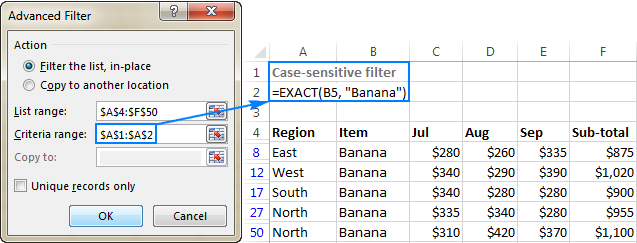Note. The above image as well all further screenshots in this tutorial show formulas in the criteria range cells solely for the sake of clarity. In your real worksheets, the formula cell should return either TRUE or FALSE, depending on whether the first row of data matches the criteria or not: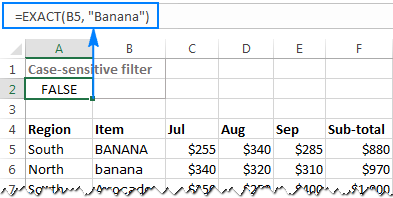### Filter values above or below average in a column

When filtering numeric values, you may often want to display only those cells that are above or below an average value in the column. For example:

To filter rows with sub-total above average, use the following formula in the criteria range:

`=F5>AVERAGE(\$F\$5:\$F\$50)`

To filter rows with sub-total below average, use the following formula:

`=F5<AVERAGE(\$F\$5:\$F\$50)`

Please pay attention that we use a relative reference to refer to the top-cell with data (F5), and absolute references to define the entire range for which you want to calculate the average, excluding the column heading (\$F\$5:\$F\$50).

The following screenshot demonstrates the above average formula in action: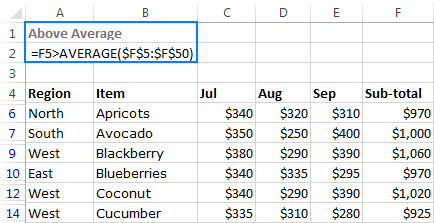Those of you who are familiar with Excel Number Filters may wonder, why would someone bother to use an advanced filter while the built-in number filters already have the Above average and Below average options? That's right, but the inbuilt Excel filters cannot be used with the OR logic!

So, to take this example further, let's filter rows where Sub-total (column F) OR September sales (column E) is above average. For this, set up the criteria range with the OR logic by entering each condition on a separate row. As the result, you will get a list of items with the above average values in either column E or F: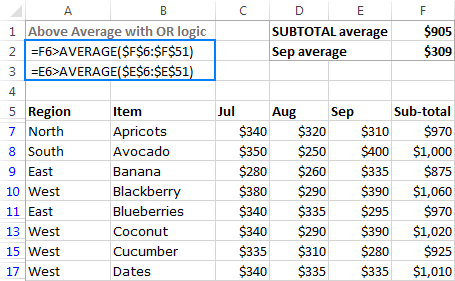### Filter rows with blanks or non-blanks

As everyone knows, Excel Filter has an inbuilt option for filtering blank cells. By selecting or deselecting the (Blanks) check box in the AutoFilter menu, you can display only those rows that have empty or non-empty cells in one or more columns. The problem is that the built-in Excel filter for blanks can work only with the AND logic.

If you want to filter blank or non-blank cells with the OR logic, or use the blank / non-blank conditions together with some other criteria, set up an advanced filter criteria range with one of the following formulas:

Filter blanks:

top_cell=""

Filter non-blanks:

top_cell<>""

#### Filtering blank cells with the OR logic

To filter rows that have a blank cell either in column A or B, or in both columns, configure the Advanced Filter criteria range in this way:

• `=A6=""`
• `=B6=""`

Where 6 is the top-most row of data.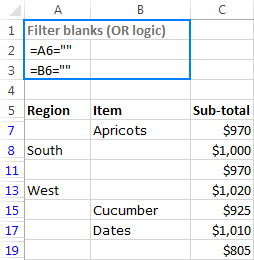#### Filtering non-blank cells with OR as wells as AND logic

To gain more understanding of how Excel's Advanced Filter works with multiple criteria, let's filter rows in our sample table with the following conditions:

• Either Region (column A) or Item (column B) should be non-blank, and
• Sub-total (column C) should be greater than 900.

To put it differently, we want to display rows that meet the following conditions:

(Subtotal>900 AND Region=non-blank) OR (Subtotal>900 AND Item=non-blank)

As you already know, in the Excel Advanced Filter criteria range, the conditions joined with the AND logic should be entered in the same row, and the conditions joined with the OR logic - on different rows:Because one criteria in this example is expressed with a formula (non-blanks) and the other includes a comparison operator (Sub-total > 900), let me remind you that:

• Criteria formed with comparison operators should have headings exactly equal to the table headings, like the Sub-total criteria in the above screenshot.
• Formula-based criteria should have either a blank heading cell or a heading that does not match any of the table headings, like the Non-blanks criteria in the above screenshot.

### How to extract top/bottom N records

As you probably know, the build-in Excel Number Filters have an option to display the top 10 or bottom 10 items. But what if you need to filter the top 3 or bottom 5 values? In this case, Excel Advanced Filter with the following formulas comes in handy:

Extract top N items:

top_cell>=LARGE(range, N)

Extract bottom N items:

top_cell<=SMALL(range, N)

For instance, to filter top 3 subtotals, create the criteria range with this formula:

`=F5>=LARGE(\$F\$5:\$F\$50,3)`

To extract bottom 3 subtotals, use this formula:

`=F5>=SMALL(\$F\$5:\$F\$50,3)`

Where F5 is the top-most cell with data in the Subtotal column (excluding the column heading).

The following screenshot shows the top 3 formula in action: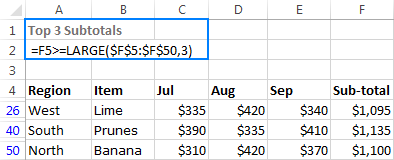Note. If the list range contains a few rows with the same values that fall into the top/bottom N list, all such rows will be displayed, like shown in the screenshot below: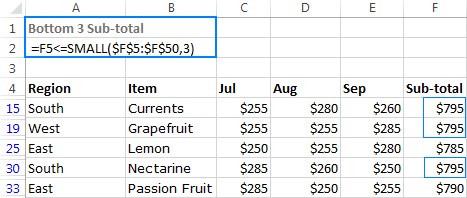### Filter for matches and differences between two columns

One of our previous articles explained a variety of ways to compare two columns in Excel and find matches and differences between them. In addition to Excel formulas, conditional formatting rules and the Duplicate Remover tool covered in the above tutorial, you can also use Excel's Advanced Filter to extract rows that have the same or different values in two or more columns. To do this, input one of the following simple formulas in the criteria range:

• Filter for matches (duplicates) in 2 columns:
`=B5=C5`
• Filter for differences (unique values) in 2 columns:
`=B5<>C5`

Where B5 and C5 are the top-most cells with data in the two columns you want to compare.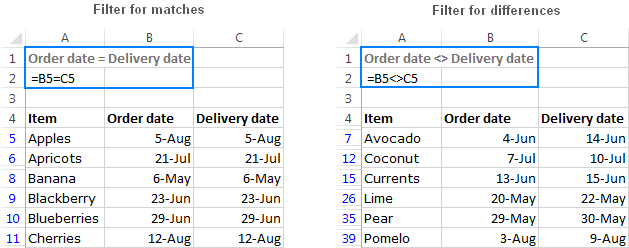Note. The Advanced Filter tool can only search for matches and differences in the same row. To find all values that are in column A but are not anywhere in column B, use this formula.

### Filter rows based on matching items in a list

Supposing you have a big table with hundreds or thousands of rows, and you received a shorter list containing only the items relevant at a given moment. The question is - how do you find all entries in your table that are or are not in the smaller list?

#### Filter rows that match items in a list

To find all items in the source table that are also present in a smaller list, using the following COUNTIF formula:

COUNTIF(list_to_match, top_data_cell)

Assuming that the smaller list is in the range D2:D7, and the table's items to be compared to that list are in column B beginning with row 10, the formula goes as follows (please notice the use of absolute and relative references):

`=COUNTIF(\$D\$2:\$D\$7,B10)`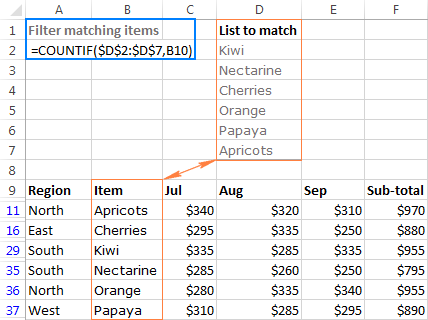Of course, you are not confined to filtering your table with just one criterion.

For instance, to filter rows matching the list, but for the North region only, enter two criteria in the same row so they will work with the AND logic:

• Region: `="=North"`
• Matching items: `=COUNTIF(\$D\$2:\$D\$7,B10)`

As you can see in the screenshot below, there are only two records in the table that match both criteria: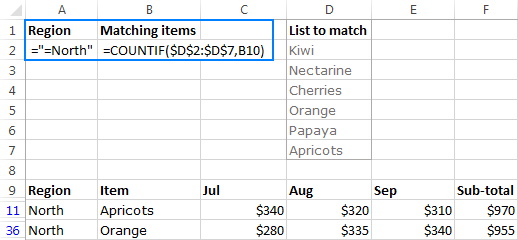Note. In this example, we use the exact match criteria for text values: `="=North"` to find only those cells that are exactly equal to the specified text. If you enter the Region criteria simply as North (without the equal sign and double quotes), Microsoft Excel will find all items that begin with the specified text, e.g. Northeast or Northwest. For more information, please see Excel Advanced Filter for text values.

#### Filter rows that do not match items in a list

To find all items in the table that are not in the smaller list, check if the result of our COUNTIF formula is equal to zero:

COUNTIF(list_to_match, top_data_cell)=0

For example, to filter the North region items in the table that do appear in the list, use the following criteria:

• Region: `="=North"`
• Non-matching items: `=COUNTIF(\$D\$2:\$D\$7,B10)=0`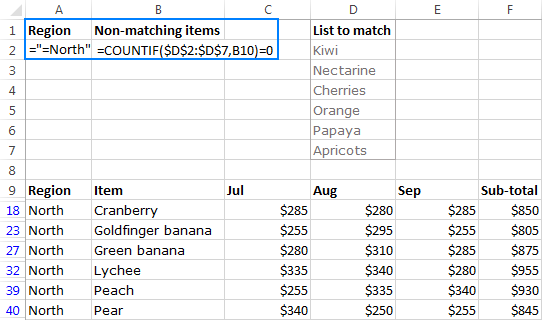Notes:

• If the list to match resides in a different worksheet, be sure to include the sheet name in the formula, e.g. `=COUNTIF(Sheet2!\$A\$2:\$A\$7,B10)`.
• If you want to extract the results to a different sheet, start the Advanced Filter from the destination sheet, as explained in How to extract filtered rows to another worksheet.

### Filter for weekends and weekdays

So far, our Advanced Filter criteria range examples have dealt mostly with numeric and text values. Now, it's time to give some clues to those of you who operate on dates.

The built-in Excel Date Filters provide a wide range of options that cover many scenarios. Many, but not all! For example, if you were given a list of dates and asked to filter weekdays and weekends, how would you go about it?

As you probably know, Microsoft Excel provides a special WEEKDAY function that returns the day of the week corresponding to a given date. And it is this function that we are going to use in the Excel Advanced Filter criteria range.

#### How to filter weekends in Excel

Keeping in mind that, in the WEEKDAY terms, 1 stands for Sunday and 6 stands for Saturday, the formula to filter weekends goes as follows:

OR(WEEKDAY(date)=7, WEEKDAY(date)=1)

In this example, we are filtering dates in column B beginning with row 5, so our Weekends formula takes the following shape:

`=OR(WEEKDAY(B5)=7, WEEKDAY(B5)=1)`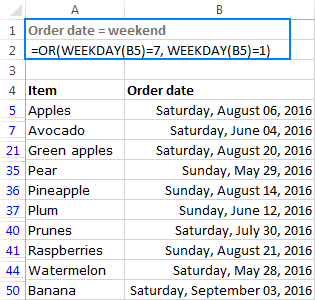#### How to filter weekdays in Excel

To filter weekdays, modify the above formula so that it will leave out 1's (Sunday) and 7's (Saturday):

AND(WEEKDAY(date)<>7, WEEKDAY(date)<>1)

For our sample table, the following formula will work a treat:

`=AND(WEEKDAY(B5)<>7, WEEKDAY(B5)<>1)`

In addition, you can add one more condition to filter out blank cells: `=B5<>""`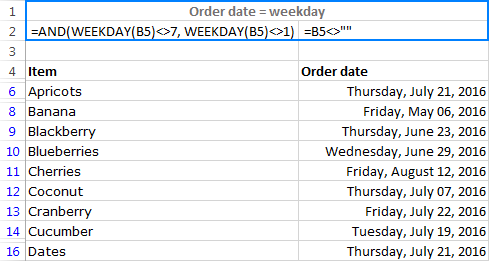To filter the dates in your worksheets in other ways, just find the relevant Date function and don't hesitate to use it in your advanced filter criteria range.

Well, this is how you use the Advanced Filter in Excel with complex criteria. Of course, your options are not limited to the examples discussed in this tutorial, our goal was just to give you a few inspirational ideas that will set you on the right track. Remembering that the road to mastery is paved with practice, you may want to download our Excel Advanced Filter examples now, and extend or reverse-engineer them for better understanding. I thank you for reading and hope to see you on our blog next week!